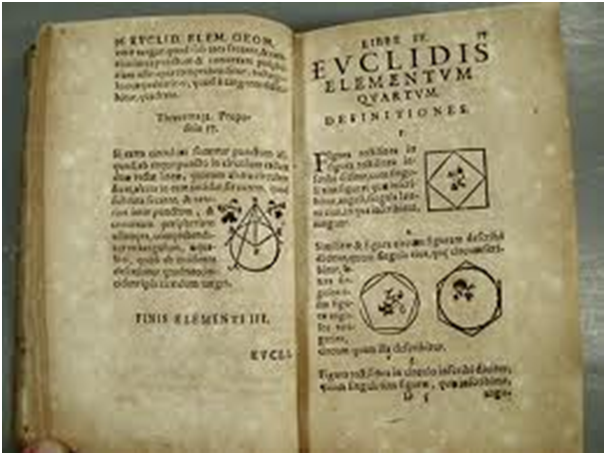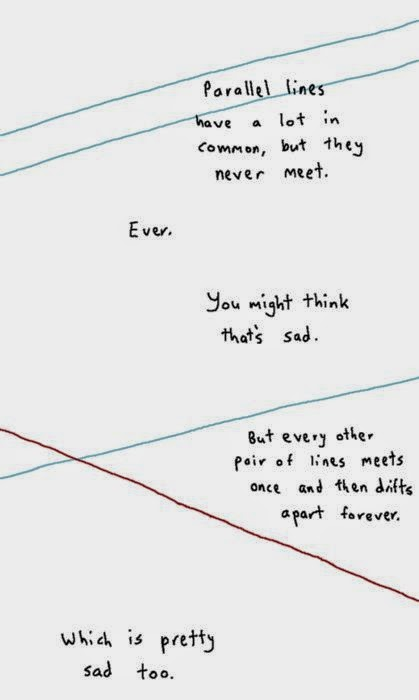## 22 May 2014

### Linear Simultaneous Equations

Solving linear simultaneous equations is rather satisfying. In this post I suggest some techniques and resources for teaching this topic.Equality and Lincoln
When I saw the film Lincoln I was struck by Abraham Lincoln’s reference to Euclid’s First Axiom ‘Things which are equal to the same thing are also equal to one another’. A well-made point in a discussion about racial equality, but also an interesting hook into a method for solving simultaneous equations such as
y = 4x + 6
y = 2x + 3
Both 4x + 6 and 2x + 3 are equal to y, therefore logically we have 4x + 6 = 2x + 3 (this is essentially just a substitution method). Why not show this clip from Lincoln in a lesson - it's a nice cross-curricular link between History and Mathematics and could even lead to a worthwhile ethical discussion (this blog gives a good explanation of Lincoln's point)

Elimination and 'SSS'
When solving simultaneous equations by elimination, my pupils find it very easy to remember the ‘SSS’ rule ie ‘Same Sign Subtract’. For example, in the equations 3x + 5y = 59; 2x + 5y = 56, this rule says that because the 5y in each equation is positive (‘same sign’), we subtract one equation from the other.

However, I noticed that when solving simultaneous equations such as -3x + 5y = 41; 2x + 5y = 56, some pupils saw that the -3x and the 2x were different signs and incorrectly decided that this meant they should add the two equations. So it’s worth clarifying which signs we are referring to in the ‘Same Sign Subtract’/’Different Sign Add’ approach.

Graphical SolutionsIt’s so important for pupils understand that whenever they solve linear simultaneous equations, they are in fact finding the point of intersection of two straight lines. It’s essential to pose a question involving parallel lines (eg solve 3x + 4y = 10; 6x + 8y = 15) and get them thinking about why there are no solutions.  I like to show them this cute picture too.

Wordy Equations
This is one of my favourite simultaneous equation questions: “A zoo has several ostriches and several giraffes. In total they have 30 eyes and 44 legs. How many ostriches and how many giraffes are in the zoo?”. Make sure you discourage pupils from using the letter O for ostrich, because it looks like a zero.

Resources
There's lots of resources online – here’s a few I’ve picked out:

1.1.